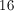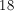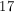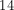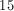## Example Questions

### Example Question #11 : How To Find Arithmetic Mean

Janice, Candice, Sharon, and Helen have an average height of 65 inches. Janice is the shortest at 55 inches, and Helen is the tallest at 70 inches. What is the average height of Candice and Sharon?

68 inches

65 inches

62.5 inches

67.5 inches

63.5 inches

67.5 inches

Explanation:

First we find the average height for Janice and Helen, (55 + 70)/2 = 62.5.   The average of the height for Candice and Sharon must complement this to achieve the overall average of 65. We can solve for their average by doing (62.5 + x)/2 = 65.

Solving this equation yields an average height of 67.5 inches.

### Example Question #12 : How To Find Arithmetic Mean

Susan got a job at a store folding the display clothes. If she folds shirts during her whole eight-hour shift, except for her thirty-minute lunch break, Susan can fold 180 shirts. How long does it take her to fold one shirt?

250 seconds

2.5 seconds

150 seconds

160 seconds

25 seconds

150 seconds

Explanation:

First you convert hours to minutes by multiplying 8 by 60, giving you 480 minutes. You then subtract her 30 minute lunch break, leaving you with 450 minutes. Then divide this by 180 shirts, giving you 2.5 minutes per shirt. Based on the available answer choices, you then multiply by 60 again to get the time in seconds, 150.

### Example Question #11 : How To Find Arithmetic Mean

Sam earned $8/hour working three eight-hour shifts, Monday through Wednesday, at her waitressing job, and earned$9.75/hour tutoring five hours a day, Friday through Sunday. Approximately, what were her daily average earnings?

$59$49

$56$58

$48 Correct answer:$56

Explanation:

First you multiply $8 by the eight-hour shift. Sam worked at her waitressing job, making$64. Then multiply that by the three days that she worked, making $192. You then multiply the$9.75 Sam made per hour by the five hours she worked by the three days she worked. This gives you $146.25. Add this to the earnings from her waitressing job, giving you$338.25. Divide this by the 6 days she worked, resulting in $56.38 a day. ### Example Question #11 : Statistics Hannah travels 321 miles on 12 gallons of gas. What is her mileage? Round to the nearest mile per gallon. Possible Answers: 23 mpg 18 mpg 35 mpg 27 mpg 25 mpg Correct answer: 27 mpg Explanation: This is a division problem: 321 miles ÷ 12 gallons = 26.75 miles per gallon, which rounds to 27 mpg. ### Example Question #11 : Statistics Derek's golf scores for 3 days were 77, 79, and 73. If his 4-day average was a 74, what was his score on the fourth day? Possible Answers: 52 67 70 71 Correct answer: 67 Explanation: (77 + 79 + 73 + x)/4 = 74 Solving for x yields 67. ### Example Question #11 : Statistics After four tests, Nick has an average of 88; what score does he need to have on the fifth test to raise his average to a 90? Possible Answers: 98 90 100 88 87 Correct answer: 98 Explanation: To have an average of 90, Nick has to have 450 points after 5 tests; with an average of 88 after 4 tests he has 352 points, which means that he needs a 98 on the fifth test to reach 450 points. ### Example Question #12 : Statistics Find the arithmetic mean of the given set of numbers: {0,12,10,8,15,5,20} Possible Answers: 7 12 11 10 8.33 Correct answer: 10 Explanation: The sum of this set of numbers is 70. There are 7 elements in the set, so 70/7 = 10 which is your mean. ### Example Question #14 : How To Find Arithmetic Mean Bryan earned$8/hr working a 6-hour shift each day, Tuesday thru Thursday, at his job as a server. He also earned $7.75/hr tutoring for a 2-hour shift each day, Friday thru Sunday. What were his daily average earnings for the 6 day period? Possible Answers:$35

$32$31

$30.75$31.75

$31.75 Explanation: We multiply the$8 by the 6 hours, and by the three 3 days that Bryan worked at his job as a server. 8 * 6 * 3 = 144

We then multiply the $7.75 an hour, by the 2 hour shift and by 3 days to get the total Bryan earned at his tutoring job. 7.75 * 2 * 3 = 46.50 We then add the totals: 144 + 46.50 = 190.50 Then divide by 6 days, getting an average of$31.75 per day.

### Example Question #19 : Statistics

History class has three major exams that make up Joe’s grade.  If he scores an 88 on the first exam, an 86 on the second exam and a 96 on the third exam, what is his average grade on all three exams?

96

88

92

86

90

90

Explanation:

Average or Mean  = Sum of the Options / # of Options

Average = (88 + 86 + 96) / 3 = 270/3 = 90

### Example Question #13 : Statistics

Find the mean: 8, 11, 17, 17, 18, 19, 22